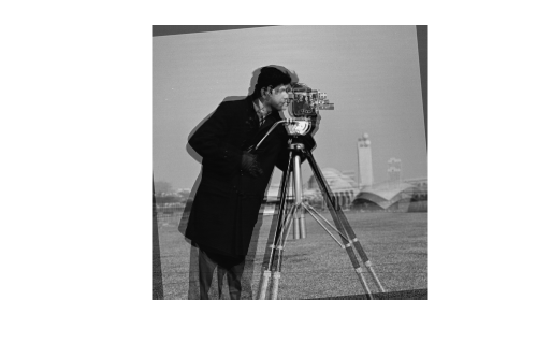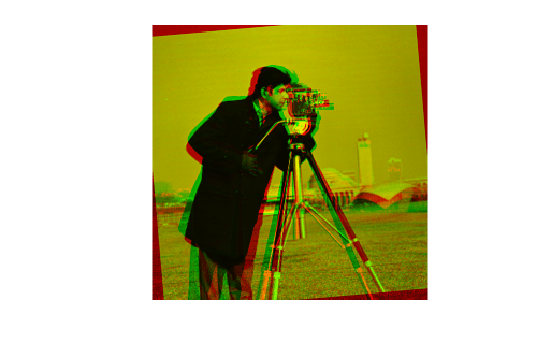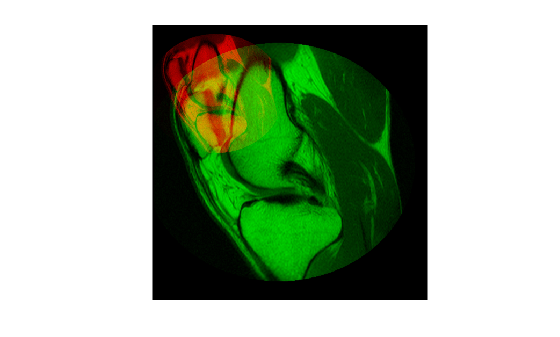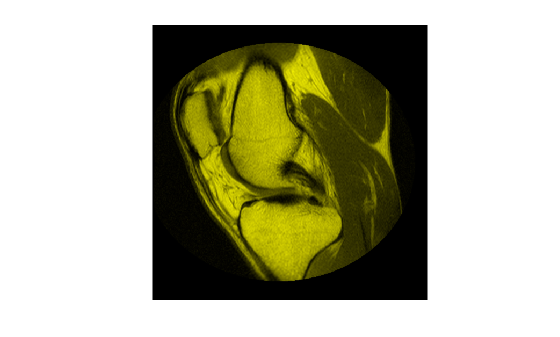# imfuse

Composite of two images

## Syntax

``C = imfuse(A,B)``
``````[C RC] = imfuse(A,RA,B,RB)``````
``C = imfuse(___,method)``
``C = imfuse(___,Name,Value)``

## Description

example

````C = imfuse(A,B)` creates a composite image from two images, `A` and `B`. If `A` and `B` are different sizes, `imfuse` pads the smaller dimensions with zeros so that both images are the same size before creating the composite. The output, `C`, is a numeric matrix containing a fused version of images `A` and `B`.```

example

``````[C RC] = imfuse(A,RA,B,RB)``` creates a composite image from two images, `A` and `B`, using the spatial referencing information provided in `RA` and `RB`. The output `RC` defines the spatial referencing information for the output fused image `C`.```

example

````C = imfuse(___,method)` uses the algorithm specified by `method`.```

example

````C = imfuse(___,Name,Value)` specifies additional options with one or more name-value arguments, using any of the previous syntaxes.```

## Examples

collapse all

Load an image into the workspace. Create a copy with a rotation offset applied.

```A = imread('cameraman.tif'); B = imrotate(A,5,'bicubic','crop');```

Create blended overlay image, scaling the intensities of `A` and `B` jointly as a single data set. View the fused image.

```C = imfuse(A,B,'blend','Scaling','joint'); imshow(C)```Save the resulting image as a `.png` file.

`imwrite(C,'my_blend_overlay.png');`

Load an image into the workspace. Create a copy and apply a rotation offset.

```A = imread('cameraman.tif'); B = imrotate(A,5,'bicubic','crop');```

Create a blended overlay image, using red for image `A`, green for image `B`, and yellow for areas of similar intensity between the two images. Then, display the overlay image.

```C = imfuse(A,B,'falsecolor','Scaling','joint','ColorChannels',[1 2 0]); imshow(C)```Save the resulting image as a `.png` file.

`imwrite(C,'my_blend_red-green.png');`

Load an image into the workspace and create a spatial referencing object associated with it.

```A = dicomread('knee1.dcm'); RA = imref2d(size(A));```

Create a second image by resizing image `A` and create a spatial referencing object associated with that image.

```B = imresize(A,2); RB = imref2d(size(B));```

Set referencing object parameters to specify the limits of the coordinates in world coordinates.

```RB.XWorldLimits = RA.XWorldLimits; RB.YWorldLimits = RA.YWorldLimits;```

Create a blended overlay image using color to indicate areas of similar intensity. This example uses red for image `A`, green for image `B`, and yellow for areas of similar intensity between the two images.

`C = imfuse(A,B,'falsecolor','Scaling','joint','ColorChannels',[1 2 0]);`

Display the fused image. Note how the images do not appear to share many areas of similar intensity. For this example, the fused image is shrunk for easier display.

```C = imresize(C,0.5); imshow(C)```Create a new fused image, this time using the spatial referencing information in `RA` and `RB`.

`[D,RD] = imfuse(A,RA,B,RB,'ColorChannels',[1 2 0]);`

Display the new fused image. In this version, the image appears yellow because the images `A` and `B` have the same extent in the world coordinate system. The images actually are aligned, even though `B` is twice the size of `A`. For this example, the fused image is shrunk for easier display.

```D = imresize(D,0.5); imshow(D)```## Input Arguments

collapse all

First image to be combined into a composite image, specified as a grayscale, truecolor, or binary image.

Data Types: `single` | `double` | `int8` | `int16` | `int32` | `int64` | `uint8` | `uint16` | `uint32` | `uint64` | `logical`

Second image to be combined into a composite image, specified as a grayscale, truecolor, or binary image.

Data Types: `single` | `double` | `int8` | `int16` | `int32` | `int64` | `uint8` | `uint16` | `uint32` | `uint64` | `logical`

Spatial referencing information associated with the input image `A`, specified as an `imref2d` object.

Spatial referencing information associated with the input image `B`, specified as an `imref2d` object.

Algorithm used to combine the images, specified as one of the following values.

MethodDescription
`"falsecolor"`Creates a composite RGB image showing `A` and `B` overlaid in different color bands. Gray regions in the composite image show where the two images have the same intensities. Magenta and green regions show where the intensities are different. This is the default method.
`"blend"`Overlays `A` and `B` using alpha blending.
`"checkerboard"`Creates an image with alternating rectangular regions from `A` and `B`.
`"diff"`Creates a difference image from `A` and `B`.
`"montage"`Puts `A` and `B` next to each other in the same image.

### Name-Value Arguments

Specify optional pairs of arguments as `Name1=Value1,...,NameN=ValueN`, where `Name` is the argument name and `Value` is the corresponding value. Name-value arguments must appear after other arguments, but the order of the pairs does not matter.

Example: `C = imfuse(A,B,Scaling="joint")` scales the intensity values of `A` and `B` together as a single data set.

Before R2021a, use commas to separate each name and value, and enclose `Name` in quotes.

Example: `C = imfuse(A,B,"Scaling","joint")` scales the intensity values of `A` and `B` together as a single data set.

Intensity scaling option, specified as one of the following values:

 `"independent"` Scales the intensity values of `A` and `B` independently when `C` is created. `"joint"` Scales the intensity values in the images jointly as if they were together in the same image. This option is useful when you want to visualize registrations of monomodal images, where one image contains fill values that are outside the dynamic range of the other image. `"none"` No additional scaling.

Output color channel for each input image, specified as one of the following values:

 `[R G B]` A three element vector that specifies which image to assign to the red, green, and blue channels. The R, G, and B values must be 1 (for the first input image), 2 (for the second input image), and 0 (for neither image). `"red-cyan"` A shortcut for the vector `[1 2 2]`, which is suitable for red/cyan stereo anaglyphs. `"green-magenta"` A shortcut for the vector `[2 1 2]`, which is a high contrast option, ideal for people with many kinds of color blindness.

## Output Arguments

collapse all

Fused image that is a composite of the input images, returned as a grayscale, truecolor, or binary image.

Data Types: `uint8`

Spatial referencing information associated with the fused image `C`, returned as an `imref2d` object.

## Tips

• Use `imfuse` to create composite visualizations that you can save to a file. Use `imshowpair` to display composite visualizations to the screen.

• When you specify spatial referencing information `RA` and `RB`, `imfuse` combines the input reference objects and obtains a bounding box that contains the world limits of both images. When the total bounding box results in non-integer pixel dimensions in world coordinates, the fused image can have extra rows or columns of black pixels. The distortion occurs because `imfuse` samples the original images according to a reduced pixel extent.

## Version History

Introduced in R2012a